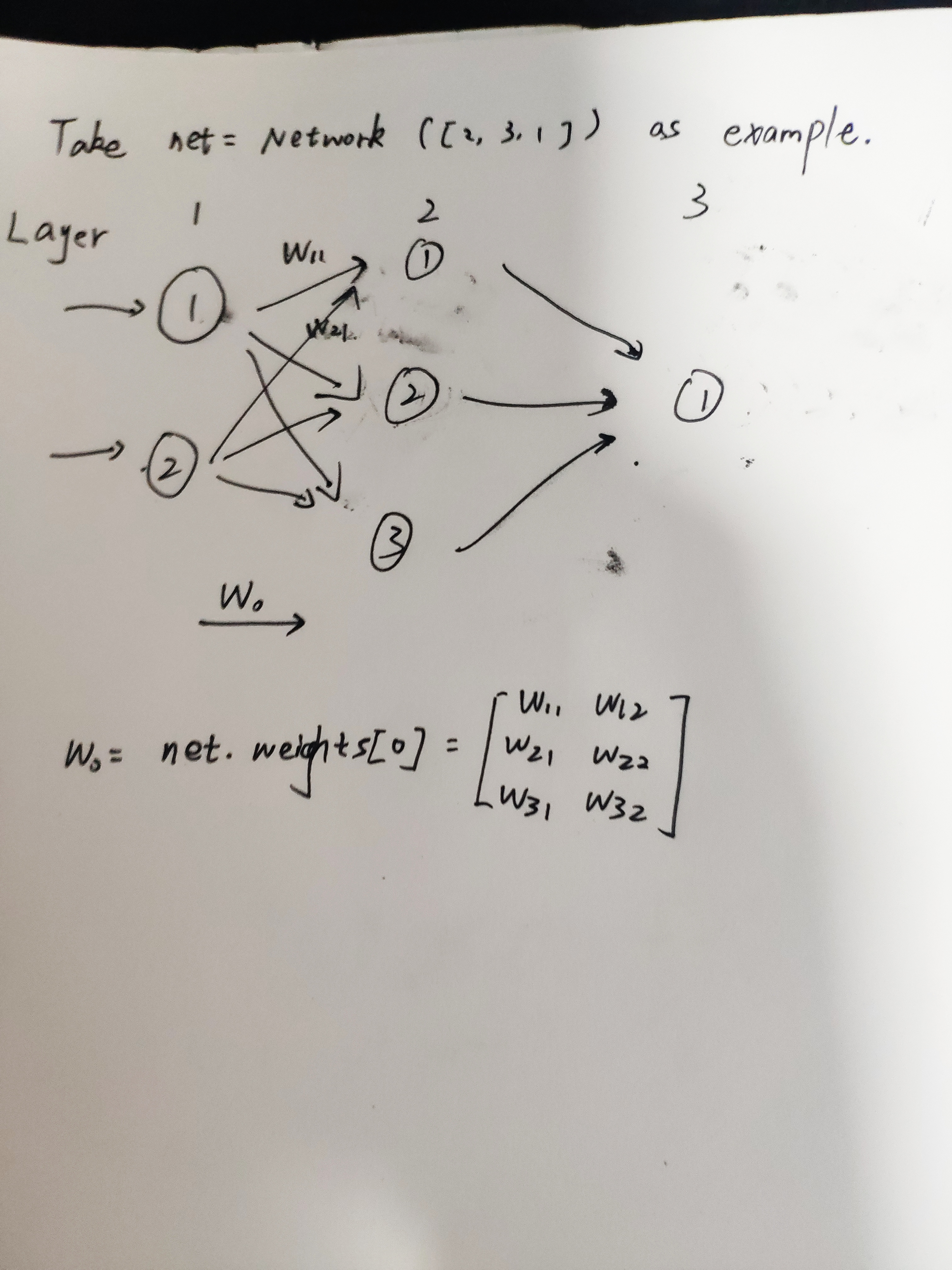# 通过MNIST手写数字识别项目的实现 了解多层感知机（MLP）的小细节

## 概念关系

### 单层感知机、多层感知机、Sigmoid神经元

• 将单层感知机构成的网络中的权重与偏置全部乘以一个正的常数c，显然神经网络的行为不会改变。
• 假如对上面所提的网络的输入已经确定，且所有神经元的激活值均不为0。现在将所有感知机换为sigmoid神经元，同样将网络中的权重与偏置乘以一个无穷大的常数c，显然调整前与调整后的网络行为不会改变。

### 神经网络的结构、隐藏层、前馈神经网络## 梯度下降法的公式描述、随机梯度下降（SGD）与学习率## 代码实现

### 初始化网络

class Network(object):
def __init__(self, sizes):
self.num_layers = len(sizes)
self.sizes = sizes
self.biases = [np.random.randn(y, 1) for y in sizes[1:]] # 随机生成偏置 注意从第二层开始才需要偏置（批注1）
self.weights = [np.random.randn(y, x)
for x, y in zip(sizes[:-1], sizes[1:])] # 随机生成权重（批注1）

np.random.randn函数将会随机生成以0为均值，1为标准差的标准正态分布数据。以后我们会接触到更合理的权重初始化方式，但如今我们先凑活用一下。
np.random.randn函数的标准形式为numpy.random.randn(d0,d1,…,dn)，没有参数时返回单个随机数，有一个参数时返回秩为1的数组，有两个及以上参数时返回对应维度的数组，可以表示向量和矩阵。参数的个数代表数组的维度，参数的数值代表对应维数的数据个数，如numpy.random.randn(3,1)表示生成一个二维数组，第一维有三个数据，第二维有一个数据。生成的数组自然也能用下标方式访问，举例如下：

>>> a=np.random.randn(3,1)
>>> print (a)
[[ 1.90478059]
[-0.96366796]
[ 1.76571214]]
>>> a
array([1.90478059])
>>> a
1.9047805907019604Wab这样的表达有点怪怪的，这样做的好处是前馈过程很容易用以下公示表达出来：${a}'=\sigma \left ( wa+b \right )$
for x, y in zip(sizes[:-1], sizes[1:])可能有点难以理解，首先zip是将两个列表合并并构成元组对，如此例：

>>> sizes = [2,3,4,5,6,7]
>>> for x, y in zip(sizes[:-1], sizes[1:]):
print (x,y)

2 3
3 4
4 5
5 6
6 7
>>> list(zip(sizes[:-1],sizes[1:])) # zip不能直接显示
[(2, 3), (3, 4), (4, 5), (5, 6), (6, 7)]### 定义sigmoid函数

def sigmoid(z):
return 1.0/(1.0+np.exp(-z))

### 在Network类中加入前馈函数

    def feedforward(self, a):
"""Return the output of the network if "a" is input."""
for b, w in zip(self.biases, self.weights):
a = sigmoid(np.dot(w, a)+b) # 注意做点积的做法
return a

### 随机梯度下降

    def SGD(self, training_data, epochs, mini_batch_size, eta,
test_data=None):
"""用小批次随机梯度下降训练神经网络  "training_data" 是元组
"(x, y)"的列表， 代表训练输入和期望输出   其他的必要参数不言自明
如果提供了test_data那么网络将会在每一次epoch后根据test_data进行评估并打印部分过程
这对跟踪进程很有用，但会拖慢训练速度 """
if test_data: n_test = len(test_data)
n = len(training_data)
for j in xrange(epochs): # 批注1
random.shuffle(training_data) #随机排序列表
mini_batches = [training_data[k:k+mini_batch_size] for k in xrange(0, n, mini_batch_size)] # 随机排序完成后取出需要的小批次作为一个列表
for mini_batch in mini_batches:
self.update_mini_batch(mini_batch, eta)
if test_data:
print "Epoch {0}: {1} / {2}".format(
j, self.evaluate(test_data), n_test)
else:
print "Epoch {0} complete".format(j)

### update_mini_batch函数

    def update_mini_batch(self, mini_batch, eta):
"""通过对一个小批次应用梯度下降（使用反向传播算法）更新网络的权重与偏置
"mini_batch" 是(x,y)元组  eta是学习率 """
nabla_b = [np.zeros(b.shape) for b in self.biases] # 用0填充与偏置维数相同的列表
nabla_w = [np.zeros(w.shape) for w in self.weights] # 用0填充与权重维数相同的列表
for x, y in mini_batch:
delta_nabla_b, delta_nabla_w = self.backprop(x, y)
nabla_b = [nb+dnb for nb, dnb in zip(nabla_b, delta_nabla_b)]
nabla_w = [nw+dnw for nw, dnw in zip(nabla_w, delta_nabla_w)]
self.weights = [w-(eta/len(mini_batch))*nw
for w, nw in zip(self.weights, nabla_w)]
self.biases = [b-(eta/len(mini_batch))*nb
for b, nb in zip(self.biases, nabla_b)]

### 加载MNIST数据集

import cPickle
import gzip
import numpy as np
"""返回一个包含(training_data, validation_data,
test_data)的元组.

training_data 是一个包含50000个2维元组的列表 (x, y)  x 是784维的numpy.ndarray包含图像信息
y 是10维numpy.ndarray代表x中装着的正确数字

validation_data 和 test_data 是包含10000个2维元组的列表 (x, y).
x 是784维numpy.ndarry包含输入图像  y是对应的分类（整数形式）

从此可以看出training_data与验证集和测试集数据结构有些许差异"""
f = gzip.open('../data/mnist.pkl.gz', 'rb')
f.close()
return (training_data, validation_data, test_data)
"""Return a tuple containing (training_data, validation_data,
test_data). Based on load_data, but the format is more
convenient for use in our implementation of neural networks.

In particular, training_data is a list containing 50,000
2-tuples (x, y).  x is a 784-dimensional numpy.ndarray
containing the input image.  y is a 10-dimensional
numpy.ndarray representing the unit vector corresponding to the
correct digit for x.

validation_data and test_data are lists containing 10,000
2-tuples (x, y).  In each case, x is a 784-dimensional
numpy.ndarry containing the input image, and y is the
corresponding classification, i.e., the digit values (integers)
corresponding to x.

Obviously, this means we're using slightly different formats for
the training data and the validation / test data.  These formats
turn out to be the most convenient for use in our neural network
code."""
training_inputs = [np.reshape(x, (784, 1)) for x in tr_d]
training_results = [vectorized_result(y) for y in tr_d]
training_data = zip(training_inputs, training_results)
validation_inputs = [np.reshape(x, (784, 1)) for x in va_d]
validation_data = zip(validation_inputs, va_d)
test_inputs = [np.reshape(x, (784, 1)) for x in te_d]
test_data = zip(test_inputs, te_d)
return (training_data, validation_data, test_data)

def vectorized_result(j):
"""Return a 10-dimensional unit vector with a 1.0 in the jth
position and zeroes elsewhere.  This is used to convert a digit
(0...9) into a corresponding desired output from the neural
network."""
e = np.zeros((10, 1))
e[j] = 1.0
return e

## 使用

git clone https://github.com/mnielsen/neural-networks-and-deep-learning.git

Python 2.7.15 (v2.7.15:ca079a3ea3, Apr 30 2018, 16:30:26) [MSC v.1500 64 bit (AMD64)] on win32
>>> training_data, validation_data, test_data = \
>>> import network
>>> net = network.Network([784, 30, 10])
>>> net.SGD(training_data, 30, 10, 3.0, test_data=test_data)
Epoch 0: 8193 / 10000
Epoch 1: 9193 / 10000
Epoch 2: 9215 / 10000
Epoch 3: 9327 / 10000
Epoch 4: 9298 / 10000
……
Epoch 17: 9489 / 10000
Epoch 18: 9498 / 10000
Epoch 19: 9504 / 10000
Epoch 20: 9495 / 10000
Epoch 21: 9498 / 10000
Epoch 22: 9487 / 10000

## 关于参数、超参数

• 参数是神经网络通过学习自己调整的，比如文中的权重与偏置，是不需要人工干预的。
• 超参数往往是训练过程的一些参数，比如文中的学习率、迭代次数等。超参数的调整有些经验主义，神经网络的调参过程几乎与神经网络的结构设计同样重要，比如迭代次数过大会引起过拟合（在训练集上准确率高，但在测试机上准确率堪忧）。说我们在本例的基础上玩一些花样：
# 花样1：修改MLP结构
>>> net = network.Network([784, 100, 10])
>>> net.SGD(training_data, 30, 10, 3.0, test_data=test_data)
# 花样2：修改学习率
>>> net = network.Network([784, 100, 10])
>>> net.SGD(training_data, 30, 10, 0.001, test_data=test_data)
Last modification：February 3rd, 2019 at 05:02 pm
If you think my article is useful to you, please feel free to appreciate